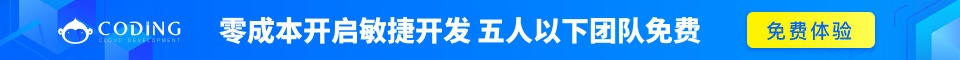# Java循环结构 – for, while 及 do…while

Java中有三种主要的循环结构：

• while循环
• do…while循环
• for循环

## while循环

while是最基本的循环，它的结构为：

```while( 布尔表达式 ) {
//循环内容
}
```

### 实例

```public class Test {
public static void main(String args[]) {
int x = 10;
while( x < 20 ) {
System.out.print("value of x : " + x );
x++;
System.out.print("\n");
}
}
}
```

```value of x : 10
value of x : 11
value of x : 12
value of x : 13
value of x : 14
value of x : 15
value of x : 16
value of x : 17
value of x : 18
value of x : 19
```

## do…while循环

do…while循环和while循环相似，不同的是，do…while循环至少会执行一次。

```do {
//代码语句
}while(布尔表达式);
```

### 实例

```public class Test {

public static void main(String args[]){
int x = 10;

do{
System.out.print("value of x : " + x );
x++;
System.out.print("\n");
}while( x < 20 );
}
}
```

```value of x : 10
value of x : 11
value of x : 12
value of x : 13
value of x : 14
value of x : 15
value of x : 16
value of x : 17
value of x : 18
value of x : 19
```

## for循环

for循环执行的次数是在执行前就确定的。语法格式如下

```for(初始化; 布尔表达式; 更新) {
//代码语句
}
```

• 最先执行初始化步骤。可以声明并初始化一个或多个循环控制变量，也可以是空语句。
• 然后，检测布尔表达式的值。如果为true，循环体被执行。如果为false，循环终止，开始执行循环体后面的语句。
• 执行一次循环后，更新循环控制变量。
• 再次检测布尔表达式。循环执行上面的过程。

### 实例

```public class Test {

public static void main(String args[]) {

for(int x = 10; x < 20; x = x+1) {
System.out.print("value of x : " + x );
System.out.print("\n");
}
}
}
```

```value of x : 10
value of x : 11
value of x : 12
value of x : 13
value of x : 14
value of x : 15
value of x : 16
value of x : 17
value of x : 18
value of x : 19
```

## Java增强for循环

Java5引入了一种主要用于数组的增强型for循环。

Java增强for循环语法格式如下:

```for(声明语句 : 表达式)
{
//代码句子
}
```

### 实例

```public class Test {

public static void main(String args[]){
int [] numbers = {10, 20, 30, 40, 50};

for(int x : numbers ){
System.out.print( x );
System.out.print(",");
}
System.out.print("\n");
String [] names ={"James", "Larry", "Tom", "Lacy"};
for( String name : names ) {
System.out.print( name );
System.out.print(",");
}
}
}
```

```10,20,30,40,50,
James,Larry,Tom,Lacy,
```

## break关键字

break主要用在循环语句或者switch语句中，用来跳出整个语句块。

break跳出最里层的循环，并且继续执行该循环下面的语句。

### 语法

break的用法很简单，就是循环结构中的一条语句：

```break;
```

### 实例

```public class Test {

public static void main(String args[]) {
int [] numbers = {10, 20, 30, 40, 50};

for(int x : numbers ) {
if( x == 30 ) {
break;
}
System.out.print( x );
System.out.print("\n");
}
}
}
```

```10
20
```

## continue关键字

continue适用于任何循环控制结构中。作用是让程序立刻跳转到下一次循环的迭代。

### 语法

continue就是循环体中一条简单的语句：

```continue;
```

### 实例

```public class Test {

public static void main(String args[]) {
int [] numbers = {10, 20, 30, 40, 50};

for(int x : numbers ) {
if( x == 30 ) {
continue;
}
System.out.print( x );
System.out.print("\n");
}
}
}
```

```10
20
40
50
```